# Blog

## Metastable ferroelectricity driven by depolarization fields in ultrathin Hf0.5Zr0.5O2

11:31 13 julio in Artículos por Website

We first present the scaling trends of HZO capacitors and the main switching characteristics of the thinnest (5 nm) ferroelectric HZO obtained. Thickness scaling and proper choice of electrode materials are both important for operation of single layer FTJ. Semiconductor bottom electrodes such as Ge or Nb-doped SrTiO3 (NSTO) are used because of their lower carrier densities compared to metals which allow for uncompensated polarization charges, the latter being very important for the FTJ functionality. Ge produces sharp, crystalline interfaces with HZO17 therefore, it is preferred over Si which typically forms low-k interfacial oxides or silicates. On the other hand, NSTO has been used successfully for the fabrication of BaTiO3 (BTO)/NSTO FTJs4, where the giant tunneling electroresistance effect was obtained by modulating the Schottky barrier thickness at the interface, for various Nb concentration18, therefore it could be a good candidate also for HZO/NSTO FTJs. HZO is deposited by plasma assisted molecular beam deposition17,19,20 and crystallization annealing is performed via Rapid Thermal Annealing (RTA)20 or Flash Lamp Annealing (FLA)17 as described elsewhere17,19,20,21 and in Methods.

The main structural characteristics of the thinnest TiN/HZO/Ge capacitor structures are shown in Fig. 1 and Supplementary Note 1. Grazing Incidence X-ray Diffraction (GIXRD) scans before (gray line, amorphous) and after annealing (blue line) are shown in Fig. 1a. The latter shows no evidence of the non-polar monoclinic phase (green lines at 28.4 and 31.7 deg22) while the main peak at 30.7 deg (Fig. 1a) is attributed to a combination of the ferroelectric orthorhombic phase Pca2123) and the tetragonal phase P42/nmc22.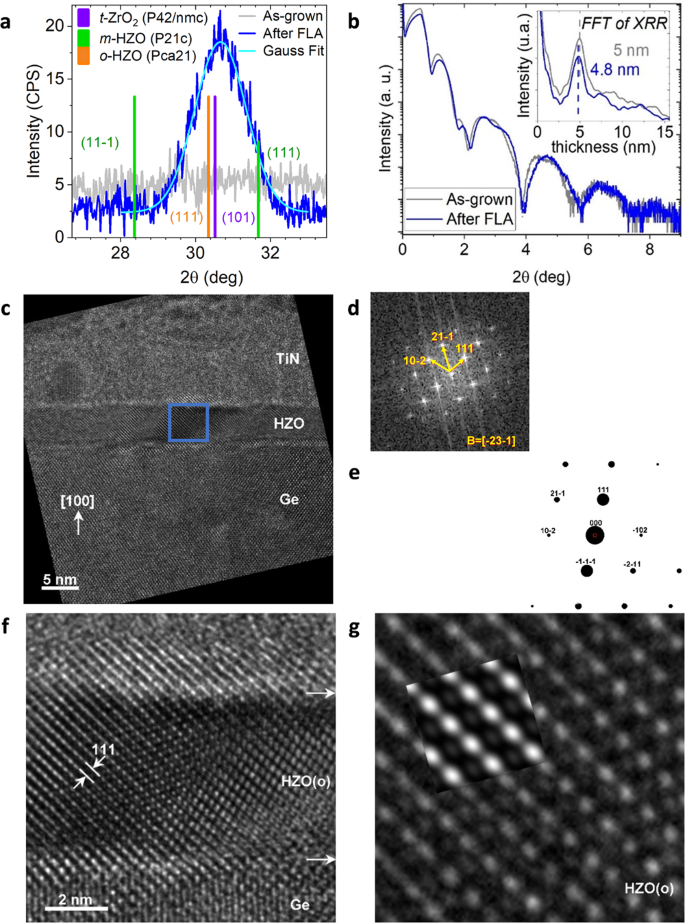Fig. 1: Microstructure of 5 nm Hf0.5Zr0.5O2 (HZO) thin films on Ge by High Resolution Transmission Electron Microscopy (HRTEM).

From the Scherrer fit of the peak at 30.7 deg, with k-factor ranging from 0.7 to 1, the average crystalline grain size along the direction ω−2θ/2 = 15 deg (approximately in-plane) is estimated to be between 4 and 5.6 nm. A HZO thickness of 5 nm is accurately measured by X ray reflectivity (Fig. 1b) and cross-sectional Transmission Electron Microscopy (TEM) (Fig. 1c and Supplementary Fig. 1). No interfacial layers at the top and bottom surface of HZO are detected by TEM (Fig. 1c and Supplementary Fig. 1), although a non-discernible amorphous TiOxNy layer at the top interface cannot be excluded. Based on filtering of the Fast Fourier Transform (FFT) pattern (Fig. 1d)), the grain size of the HZO polycrystalline film is estimated between 5 nm and 20 nm (Supplementary Fig. 1), noting that the lower limit is compatible with the GIXRD results discussed above. Based on FFT (Fig. 1d, e) and real image (Fig. 1g) simulations, the observed nanocrystallites (Fig. 1f) are compatible with the ferroelectric orthorhombic phase Pac21. However, the distinction between orthorhombic and tetragonal at the macroscale based on GIXRD (Fig. 1a) is difficult. It is anticipated that possible tetragonal phase will be present in rather small quantities since, after wake-up, the remanent polarization Pr is high (>20 μC cm−2) (see Supplementary Note 2 and discussion below) indicating the dominance of the ferroelectric orthorhombic phase.

Figure 2 shows the evolution of the switching characteristics as a function of decreasing HZO thickness for Ge and NSTO Metal Ferroelectric Semiconductor (MFS) capacitors. The general trend is that thick HZO capacitors show full ferroelectric hysteresis loops in the pristine state, while as the thickness is reduced, the hysteresis loops become distorted and at 5 nm, they are pinched (AFE-like) yielding low remanent polarization. Full hysteresis loops are recovered (“waked-up”) after field cycling while the two switching peaks in the displacement current nearly merge.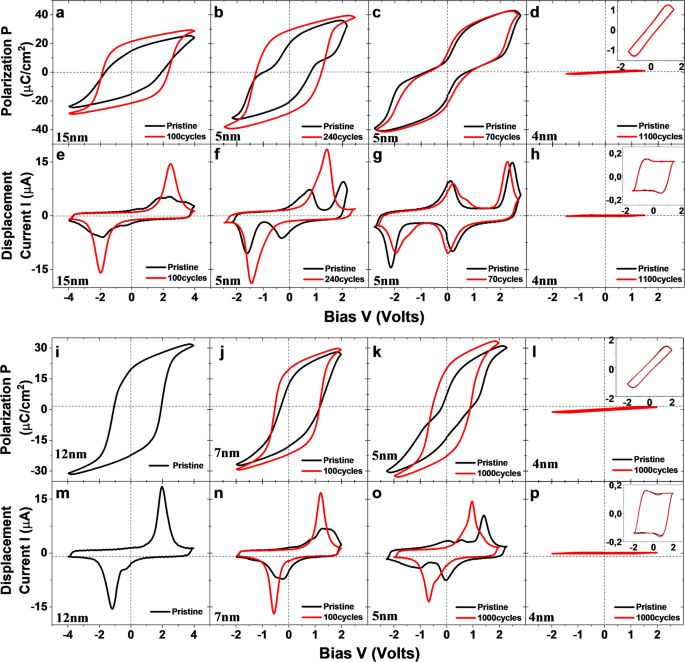Fig. 2: Evolution of ferroelectric hysteresis of Hf0.5Zr0.5O2 with Hf0.5Zr0.5O2 thickness scaling.

For thick HZO films (Fig. 2a and b), only few cycles are needed but for thinner films, larger number of cycles are required to wake-up the devices. In some cases (as in Fig. 2c, g), the devices are not affected by cycling at all and remain pinched. It should be noted that for 4 nm HZO and thinner (Fig. 2d, h, l, p), ferroelectricity becomes obsolete and HZO behaves like a normal dielectric.

The waked up devices down to the thinnest 5 nm HZO show high remanent polarization Pr > 20 μC cm−2 after correction, with only small parasitic (non-switchable) polarization (see PUND measurements in Supplementary Fig. 2) and a low coercive voltage Vc ~ 1 V. These performance characteristics are very promising for low voltage/low power FTJ memory devices. Moreover, the high Pr indicates the predominance of the ferroelectric orthorhombic phase as already mentioned above.

The capacitance-voltage measurements on p-Ge/HZO/TiN capacitors at 1 kHz are shown in Fig. 3 featuring the characteristic “butterfly” structure due to ferroelectricity in HZO. Due to the “butterfly” structure which masks the capacitance variations with bias, and the low energy gap of Ge (consequently the high minority carrier concentration) the C-V at room temperature looks more like an MFM capacitor rather than MFS. Note however, that at lower temperatures where the minority carrier concentration is dramatically reduced, a more typical MOS-like behavior is recovered with a clear distinction between accumulation and depletion17. The accumulation capacitance Cacc (measured at > −2V) of the 5 and 15 nm capacitors are measured to be 3.1 and 1.7 μF cm−2, respectively. If a single layer HZO is assumed, the measured Cacc yields a value of HZO relative dielectric constant εF = 18 which is not compatible with the orthorhombic ferroelectric phase, since the latter has an expected value of εF in the range between 30 and 40 [refs. 20,24,25,26,27,28]. It is therefore necessary to consider an interfacial capacitance Cint in series with the FE capacitance CF in order to account for the measured Cacc. Taking into account both 5 nm and 15 nm capacitors and by assuming the same Cint and εF, values of Cint ~ 6 μF cm−2 and εF ~ 39 are extracted. This value of Cint is considerably smaller than the values of 30–110 μF cm−2 reported for Metal-Ferroelectric-Metal (MFM) capacitors27,29 with very thin or no interfacial layers.

In our case, Cint could be dominated from the semiconductor region near the interface due to the finite screening length l. Using an expression3 for $$l=\frac{{{{{{\rm{h}}}}}}}{q}* \sqrt{\frac{{\varepsilon }_{0}{\varepsilon }_{{Ge}}}{3{m}^{* }}}* {\left(\frac{3}{8\pi }\right)}^{\frac{1}{3}}* {\left(\frac{1}{{n}_{h}}\right)}^{\frac{1}{6}}$$ (h is the Planck constant and q is the electron charge), derived for metallic systems and assuming a hole concentration nh = 1.6*1017 cm−3, effective mass m* = 0.29me30 and εs (Ge) = 16, a value of l ~ 3.5 nm and a corresponding capacitance Cs~ε0εs/l~3.6 μF cm−2 are estimated. Although Cs is only a rough estimate of the semiconductor capacitance, it does not differ much from the Cint derived from capacitance measurements. In fact, a capacitance Cit due to interface defect charging could be added in parallel to Cs so the total capacitance Cint = Cs + Cit could better match the measured value of 6 μF cm−2 (see above). In conclusion, the semiconductor capacitance, including possible contribution from interface defects, could account for the rather small value of measured Cint even in the absence of interfacial (dead) dielectric layers.

### Metastable ferroelectricity and pinched pristine hysteresis curves

To analyze the switching characteristics of the thin HZO MFS capacitors,

we assume a 1st order ferroelectric with Gibbs free energy [Eq. 1].

$$G=\frac{1}{2}a{P}^{2}+\frac{1}{4}\beta {P}^{4}+\frac{1}{6}\gamma {P}^{6}-{E}_{F}P$$

(1)

where P is the polarization order parameter and α < 0, β < 0 and γ > 0 are the Landau coefficients. From simple electrostatics (see Supplementary Note 3, for details and refs. 31,32), the electric field EF in the ferroelectric is given by

$${E}_{F}=\frac{V}{{t}_{F}}{\left[1+\frac{{C}_{F}}{{C}_{{int}}}\right]}^{-1}-\frac{P+\sigma }{{\varepsilon }_{0}{\varepsilon }_{F}}{\left[1+\frac{{C}_{{int}}}{{C}_{F}}\right]}^{-1}$$

(2)

Where V is the applied bias on the MFS, and CF, Cint are the capacitances of the ferroelectric and interfacial layers, respectively and tF is the FE thickness. The first term in the right-hand side of the equation accounts for the reduction of the volage in the ferroelectric by a factor $${\left[1+\frac{{C}_{F}}{{C}_{{int}}}\right]}^{-1}$$, since part of the voltage drops on interfacial (dead layers), or, on the semiconductor near the interface. The second term in the right-hand side of Eq. (2) is the electric field built as a result of uncompensated charge. The surface charge σ is the charge trapped at pre-existing defects at the interface; it has a sign opposite to that of P and assists in the compensation of polarization charges thus stabilizing P. Charge σ becomes important only during field cycling (wake-up) when charge injection and trapping occur and it will be discussed in subsequent sections. Here in this section, we study the pristine case where σ ≈ 0. It should be noted that when V = 0, then P = Ps (spontaneous polarization) and EF=Edep= $$-\frac{{P}_{s}}{{\varepsilon }_{0}{\varepsilon }_{F}}{\left[1+\frac{{C}_{{int}}}{{C}_{F}}\right]}^{-1}$$, that is the DF.

Using (1) and (2) with σ ≈ 0, the FE thickness dependent expression for G becomes

$$G= \frac{1}{2}a{P}^{2}+\frac{1}{4}\beta {P}^{4}+\frac{1}{6}\gamma {P}^{6}+\,\frac{1}{{\varepsilon }_{0}{\varepsilon }_{F}}{\left[1+\frac{{t}_{F}{C}_{{int}}}{{\varepsilon }_{0}{\varepsilon }_{F}}\right]}^{-1}{P}^{2}\\ -\frac{V}{{t}_{F}}{\left[1+\frac{{\varepsilon }_{0}{\varepsilon }_{F}}{{t}_{F}{C}_{{int}}}\right]}^{-1}P$$

(3)

In the last equation, CF has been expressed as CF=ε0εF/tF, where tF is the FE thickness. A corresponding expression for Cint cannot be given due to the lack of knowledge about the interfacial (dead) layer characteristics. Instead, experimental Cint values are deduced from CV analysis (see Fig. 3 and related discussion above) which yield Cint.

The polarization switching dynamics under the influence of a time varying bias V are described by the Landau-Khalatnikov (L-K) equation $$\delta \frac{{dP}}{{dt}}=-\frac{{dG}}{{dP}}$$ 33,34 where δ is the kinetic (or viscosity) coefficient. Using Gibbs free energy from Eq. (3), the L-K equation becomes:

$$\delta \frac{{dP}}{{dt}}=\,-\left[\left(a+\,\frac{2}{{\varepsilon }_{0}{\varepsilon }_{F}}{\left[1+\frac{{t}_{F}{C}_{{int}}}{{\varepsilon }_{0}{\varepsilon }_{F}}\right]}^{-1}\right)P+\beta {P}^{3}+\gamma {P}^{5}-\frac{V}{{t}_{F}}{\left[1+\frac{{\varepsilon }_{0}{\varepsilon }_{F}}{{t}_{F}{C}_{{int}}}\right]}^{-1}\right]$$

(4)

The L-K Eq. (4) is solved by applying time-varying, triangular waveforms for V (see Supplementary Note 4, Supplementary Note 5) to simulate the experimental P-V curve while equation $$J=\frac{{dD}}{{dt}}={\varepsilon }_{0}\frac{d{\rm E}}{{dt}}+\frac{{dP}}{{dt}}$$ is complementarily used to calculate the displacement current JV curves. The simulated curves (Fig. 4a and b) using a kinetic coefficient δ = 2.5*106 s cm F−1 and Landau parameters α = −23*1010 cm F−1, β = −21.4*1019 cm5 F−1 Cb−2 and γ = 15.5*1028 cm9 F−1 Cb−4, are in semiquantitative agreement with the experiment.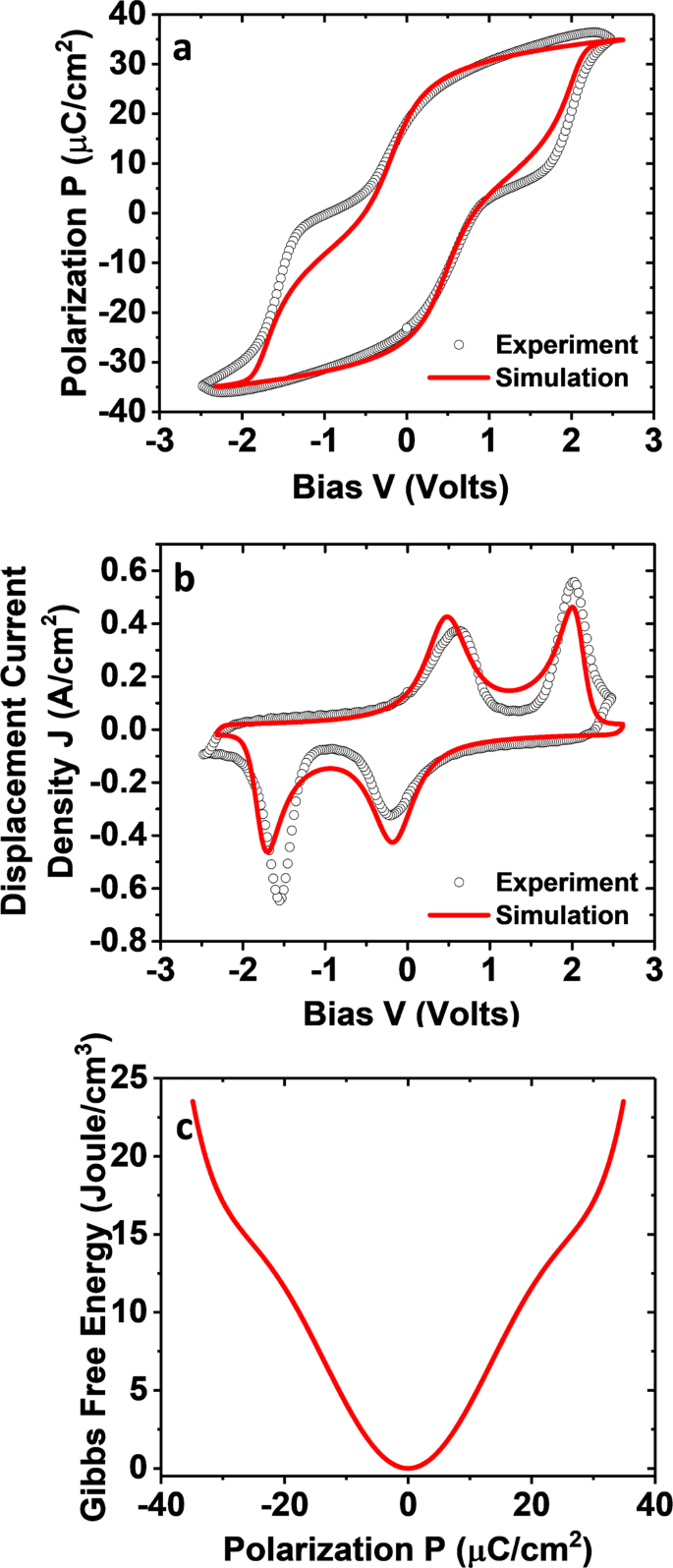Fig. 4: Comparison between experimental and modeling data of 5 nm Hf0.5Zr0.5O2 thin film.

The deviations are partly due to non-idealities of the experimental curves. A small offset in the voltage (~0.15 V) was set in the calculations in order to account for rigid shifts to higher bias due to small workfuncion differences between top TiN and bottom Ge electrodes. The simplified phenomenological LGD model which ignores extrinsic factors affecting switching dynamics (see discussion section) also accounts for the discrepancies between for the experimental and simulated curves.

At a fixed FE thickness of tF = 5 nm, Cint and εF are the two parameters which control the depolarization ~P2 term in Eq. (3), hence the pinching of the hysteresis curve. It should be emphasized that the pinching characteristics (reduced Pr) are best simulated with Cint = 5.5 μF cm−2 and εF = 39 which compare well with the values of Cint and εF obtained from the analysis of the C-V measurements (Fig. 3). Our modeling indicates that the positive depolarization term (~P2) in the Gibbs free energy counteracts the negative ¼ βP4 (and the negative ½ αP2) term and results in the triple potential well (Fig. 4c) which describes a metastable HZO ferroelectric. The stable paraelectric phase (P = 0) is then responsible for the pinching of the P-V hysteresis reducing the remanent polarization to near zero values. More insights are given in the discussion and conclusions section below.

To better illustrate the influence of Cint on the ferroelectric stability and the switching characteristics, the Gibbs free energy landscapes and the P-V curves for two different Cint values of 10 and 20 μF/cm2 are given in Fig. 5. When Cint is has an intermediate value ~10 μF cm−2, the 6 nm film shows metastable ferroelectricity (pink curve) and a weakly pinched loop with reduced Pr. The 4 and 2 nm HZO show very small remanent polarization and an ordinary dielectric behavior. When Cint becomes larger (20 μF cm−2), the situation is improved since the 6 nm HZO has stable ferroelectricity and a fully open hysteresis loop with high Pr.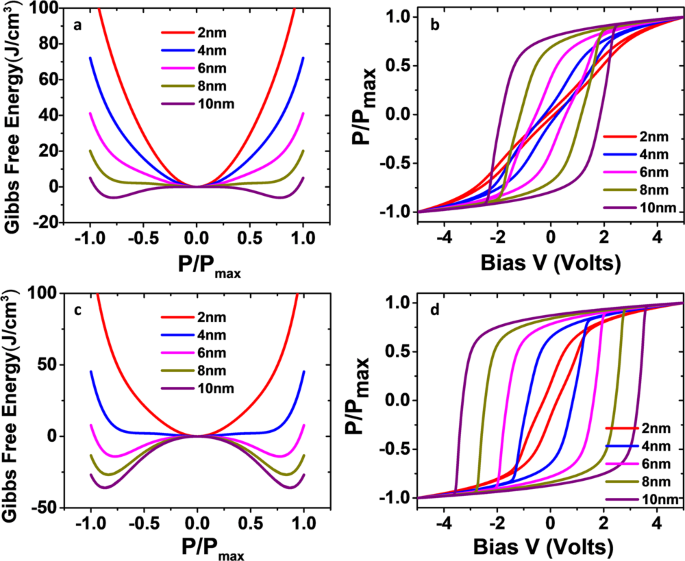Fig. 5: Interfacial capacitance (Cint) effect on Gibbs free energy landscape and P–V hysteresis curves.

The 4 nm HZO is marginally stable ferroelectric (blue curve), with a nearly open ferroelectric loop and only the very thin 2 nm film shows metastable ferroelectricity resembling an ordinary dielectric behavior. Therefore, it can be concluded that by increasing the Cint above 20 μF cm−2 it is possible to drive the system to a stable ferroelectric state even for very thin films <4 nm.

In MFM capacitors, an infinitely high Cint corresponds to perfect metal electrodes forming sharp interfaces27,29 with the HZO (without dead layers). However as already briefly mentioned in the introduction, at least one of the electrodes should be less than perfect to allow for incomplete compensation of the polarization charge, which is essential for the operation of ultrathin FTJs. Therefore, in most cases of interest related to FTJs, Cint is expected to be small resulting in loop pinching. Nevertheless, severely pinched loops at small thickness <6 nm can be recovered by injecting charge during field cycling, as described in the following section.

### Field cycling (“wake-up”) in the framework of Landau-Ginzburg-Devonshire theory

Charge injection35 can occur during field cycling since pre-existing defect states are filled with trapped electrons which could tunnel through interfacial layer barriers, if such barriers exist. This mechanism (Fig. 6) provides efficient screening of polarization charges mitigating Edep thus stabilizing polarization. Both, polarization P and applied bias V, control the states available for trapping and consequently the total trapped charge.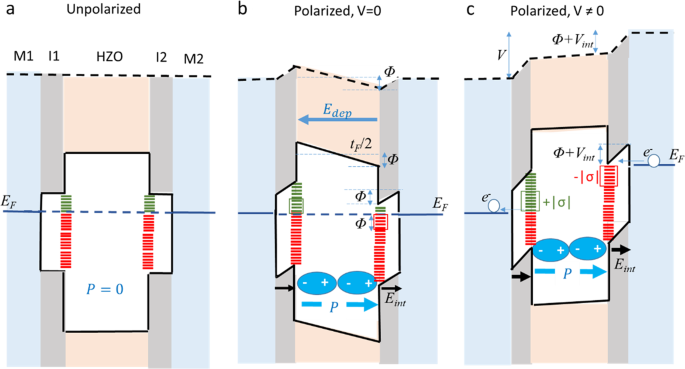Fig. 6: Schematic illustration of trap filling controlled by Polarization P and Voltage V. Depicts an idealized Hf0.5Zr0.5O2 (HZO) Metal-Insulator-Ferroelectric-Insulator-Metal (MIFIM) capacitor with symmetric interfacial layers and interface defect density, uniformly distributed in energy.

Although the analysis presented below is made for the case of identical I and M-layers on either side (Fig. 6a), with appropriate adaptations it could be valid more generally for a structure with different insulator (I) and metal (M) layers on the opposite sides including the cases where one I-layer is missing or one M-layer is a semiconductor. As a first approximation, a constant energy density of states is considered here.

Once established (Fig. 6b), the polarization lowers the potential for electrons at the interface by an amount Φ proportional to P according to Φ = (tF/2)|Edep | = tFP/2ε0εF(1 + Cint/CF). As a consequence, a portion of interface states within an energy ΔEΦ =  moves below EF and becomes accessible to electrons for trapping.

An applied external bias V further lowers the potential (Fig. 6c) at the interface by an amount Vint = V/2(1 + Cint/CF) making more states accessible to electrons in an energy interval ΔEV = eVint. The total states accessible for charge trapping are within the energy interval ΔΕ = e(Φ + Vint) therefore, the total trapped charge, in absolute value, is

$$\left|\sigma \right|=e{n}_{{tr}}\triangle E=\frac{1}{2}e{n}_{{tr}}{\left(1+\frac{{C}_{{int}}}{{C}_{F}}\right)}^{-1}\left(\frac{{t}_{F}}{{\varepsilon }_{0}{\varepsilon }_{F}}P+V\right)$$

(5)

In Eq. (5), ntr is the trapped electrons density per unit energy which is determined by solving the Shockley Read Hall36,37 rate equation ∂ntr/∂t = cn(Ditntr) −enntr. Dit is the density of interface states per unit energy and cn, en are the capture and emission rates38.

In Fig. 7a the trapped carrier density per unit energy is plotted as a function of field cycles and for two different Dit, while the details near the origin are shown in Fig. 7b. The carriers are captured in the first half of the cycle, while a smaller portion is emitted back to the electrodes during the second half of the cycle. A positive balance of carriers remains captured after each complete cycle so gradually the trapped density increases and finally saturates at a value that depends on the Dit: a larger Dit allows for more trapped charge density as can be seen from the comparison between the green and red curves in Fig. 7a.

The corresponding total charge density σ captured in all energy levels made available by P and V is calculated using Eq. (5) and is plotted in Fig. 7c. The detail at the origin in Fig. 7d shows the different contributions from the polarization P (red) and the bias V (blue) according to Eq. (5) as well as the total σ (black). It should be noted that σ has always opposite sign to both, the polarization charges and the bias therefore it changes sign in each half period when P and V also change sign. The two interfaces have charges of opposite sign which alternates in each half period. Setting a relatively high value of Dit  ~ 1.4 × 1013 eV−1 cm−2, the calculated charge is accumulated to both interfaces increasing in amplitude and saturating after about 160 cycles at a value of about 10 μC cm−2 which is a substantial fraction of the polarization charge (~20 μC cm−2) (Fig. 7c).

This indicates that after cycling, the polarization charge is sufficiently screened, thus minimizing the DF and stabilizing ferroelectricity.

A large Dit determines the screening efficiency of the polarization charges and ensures the opening of the ferroelectric loop. On the other hand, en (consequently cn) determine how fast the traps are filled up to the saturation value. Higher en (and cn) ensures faster filling so wake-up and loop opening is easier occurring only after a small number of cycles.

Introducing the contribution of trapped charge σ (Eq. (5) and Fig. 7c)) in the expression for the electric field EF (Eq. (2)), additional P2 and P terms appear in the Gibbs free energy (Eq. (3)) and L-K equations (Eq. (4), and Supplementary Fig. 5)), respectively, that counteract the depolarization terms and make ferroelectricity stable again alleviating the pinched characteristics after cycling is completed. The results are summarized in Fig. 8 above.